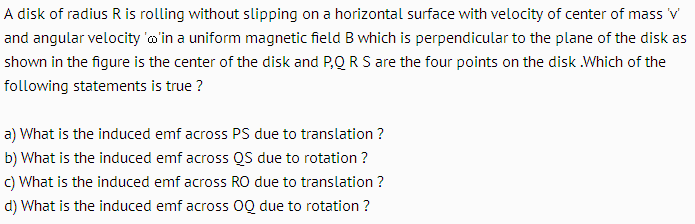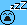# EMF induced in a rolling disk

• Vibhor
In summary, the emf induced across PS can be found by thinking as if a rod PS is rotating and translating . The component of velocity of rod perpendicular to its length is vsin45° = v/√2 . The length PS = √2R . Hence emf induced would be B(v/√2)(√2R) = BvR.f

## Homework Statement## The Attempt at a Solution

O is the center of the disk

Part a) The emf induced across PS can be found by thinking as if a rod PS is rotating and translating .

The component of velocity of rod perpendicular to its length is vsin45° = v/√2 . The length PS = √2R . Hence emf induced would be B(v/√2)(√2R) = BvR.

Is that correct ?

Thanks

#### Attachments

Last edited:
I believe that's right. I assume "due to translation" means to treat the disk as sliding along the surface with speed v with no rotation, while "due to rotation" means to keep the center of the disk at rest and rotate the disk about the center with angular speed ω.

•Vibhor
Ok.

But what if the question asked "What is the emf induced in PS due to rotation ?" . I found this a bit difficult .

For this I instead took the bottommost point as instantaneous axis and considered rod PS in pure rotation . Then I applied the result ##\frac{1}{2}Bωl^2## to this and found total induced emf = ##\frac{1}{2}B( \frac{v}{R})(√2R)^2## = BvR . On comparing this "total induced emf" with the previous result i.e induced emf due to translation , I concluded that emf induced in rod PS due to rotation is 0 .

Is this result correct ?

You should be able to argue that the emf for PS is zero "due to rotation" if "due to rotation" means rotation about the center.

•Vibhor
Do you find my above reasoning inadequate ?
You should be able to argue that the emf for PS is zero "due to rotation" if "due to rotation" means rotation about the center.

Are you suggesting that I should imagine the rod PS in pure rotation about the center O and see if it cuts any magnetic field lines ?

Do you find my above reasoning inadequate ?
No, your reasoning is correct. I was just saying that you can see that the emf due to rotation about the center is zero more directly.

Are you suggesting that I should imagine the rod PS in pure rotation about the center O
Yes
and see if it cuts any magnetic field lines ?
More specifically, consider the emf for small sections of PS. You shouldn't need to actually carry out an integration to deduce the total emf.

•Vibhor
Ok .

So , if a rod translates and rotates then Is induced emf across the rod = emf induced due to translation + emf induced due to rotation ??

Can I think of it as a general result ?

So , if a rod translates and rotates then Is induced emf across the rod = emf induced due to translation + emf induced due to rotation ??

Can I think of it as a general result ?
Yes. The velocity of any element of the rod is a sum of the velocity due to translation and the velocity due to rotation.

(til tomorrow)

•Vibhor
Ok .

The line PS divides the disk into two unequal sized circular segments ,say I and II .

When we say induced emf across PS is BvR, we are basically dealing with two parallel EMF's ,each of magnitude BvR , between points P and S . These emfs are due to portion I and II of the disk . The equivalent of two parallel EMF's of magnitude BvR , across PS , is BvR .

Makes sense ?

You should be able to argue that the emf for PS is zero "due to rotation" if "due to rotation" means rotation about the center.

More specifically, consider the emf for small sections of PS. You shouldn't need to actually carry out an integration to deduce the total emf.

This is how I tried to reason it out .

There would be only one point on the rod (call it M) which would be moving at right angles to the line joining the point to the center O . 'M' is also the mid point of the rod . In other words this point would have velocity directed along the length of the rod . Except this point , every point on PS will have a velocity component perpendicular to the length of the rod .

Now , consider a tiny length AB in the portion PM of the rod .This will have a component of velocity vP perpendicular to the length PS , but directed away from center O . Corresponding to this AB there will be a tiny length CD in MS part of the rod .This will also have a component of velocity vP perpendicular to the length PS , but directed towards center O .

The two tiny EMF's in AB and CD wil cancel each other as far as induced emf across PS is concerned . Similarly EMF in each part of PM will cancel that generated in MS . The result is that emf induced in PS due to rotation is 0 .

Is this reasoning fine ?

There would be only one point on the rod (call it M) which would be moving at right angles to the line joining the point to the center O . 'M' is also the mid point of the rod . In other words this point would have velocity directed along the length of the rod . Except this point , every point on PS will have a velocity component perpendicular to the length of the rod .

Now , consider a tiny length AB in the portion PM of the rod .This will have a component of velocity vP perpendicular to the length PS , but directed away from center O . Corresponding to this AB there will be a tiny length CD in MS part of the rod .This will also have a component of velocity vP perpendicular to the length PS , but directed towards center O .

The two tiny EMF's in AB and CD wil cancel each other as far as induced emf across PS is concerned . Similarly EMF in each part of PM will cancel that generated in MS . The result is that emf induced in PS due to rotation is 0 .

Is this reasoning fine ?
Yes, very nice.

•Vibhor
Ok . Is post#10 correct ??

The line PS divides the disk into two unequal sized circular segments ,say I and II .

When we say induced emf across PS is BvR, we are basically dealing with two parallel EMF's ,each of magnitude BvR , between points P and S . These emfs are due to portion I and II of the disk . The equivalent of two parallel EMF's of magnitude BvR , across PS , is BvR .

Makes sense ?
Yes, I believe it's ok to think of it that way. It's like the picture shown below where you have rods moving through a B field into the page (not shown). In the figure on the left, you can think of the emf from A to B of the wide rod as due to two parallel emf's by dividing the rod into two halves as shown. Likewise, in the figure on the right, the emf of the long rod from A to C can be thought of as two emf's in series (A to B plus B to C).

#### Attachments

•Vibhor
Thanks a lot .